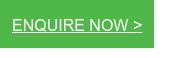# What is U-Value vs R-Value When it Comes to Door Insulation?

To understand how insulation works, it is first necessary to explain the different ways heat flows through a construction. Physically, heat always moves from areas of high temperature to those at a lower temperature, which is why, in the cold external temperatures of winter, the warmth inside a building will try to escape through walls, doors, windows, roof and floor. In order to perform effectively, an insulation material must reduce this heat flow.Thermal Resistance (R-Value)

The thermal resistance rating or R-Value is the measure used most commonly in the building and construction industry to determine a specific material’s ability to resist the transfer of heat, and is the inverse to thermal conductivity (k-value).

The higher the R-Value the better thermal resistance the product will provide, and the more energy you will save. Units: m2K/W.

R-Value = Thickness (m) / Thermal conductivity (W/mK)

Thermal Transmittance (U-Value)

Thermal transmittance, also known as U-value, is generally used to rate door or window units, and  is the rate of transfer of heat through one square metre of a structure divided by the difference in temperature across the structure. It is expressed in watts per metres squared kelvin, or W/m²K. The better-insulated a door is, the lower the U-value will be.

In its simplest form, a U-value is calculated by establishing the thermal resistance of each layer in the construction element and adding them together to provide a total resistance (TR) value. The U-value is  calculated from the reciprocal (that is, U = 1/R and R = 1/U) of the combined resistances of the materials in the element, including any airspaces and surface resistance values.

For instance an door with a total thermal resistance (R-Value) of 3.50m2K/W would have a U-value of:

1  /  3.5 = 0.29W/m2K

A high R-value means a low U-value but the real differences between them are far more complex.

U-value is more of an engineering term which describes thermal performance. It has traditionally been applied to materials such as door systems which are made up of a number of different materials. R-value is usually used in reference to construction components that are made up of only one material.

U-values represent the transfer of energy through conduction and radiation while R-value only represents resistance to heat transfer.

Top 3 Tips:

• Higher numbers are good when comparing the Thermal Resistance and R-Values of products.
• Low numbers are good when comparing U-Values.
• The U-Value is the most accurate way to judge a material’s insulating ability, taking into account all the different ways in which heat loss occurs, however it is more difficult to calculate.

Reach out to our team if you have any questions, and check out our range of insulated freezer doors.